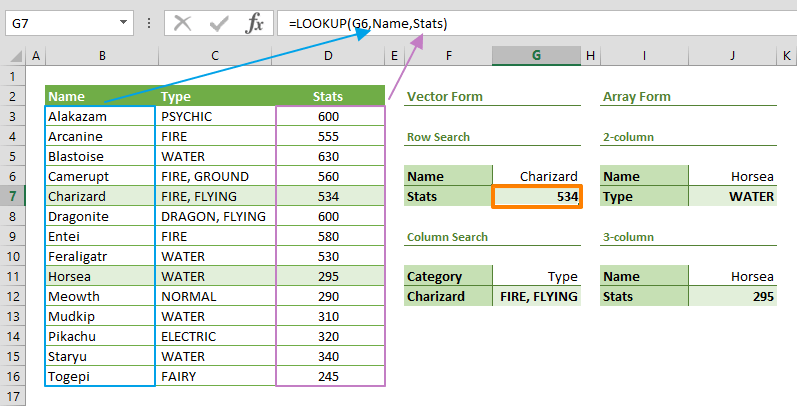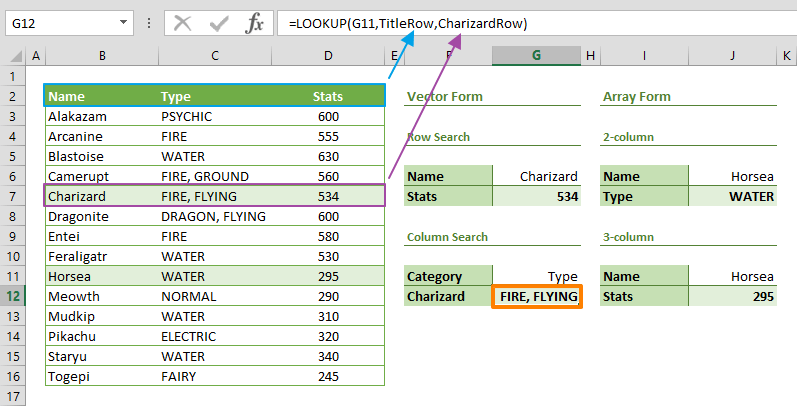The Excel LOOKUP is a Lookup & Reference formula that can search a value inside a column or row, and return a matching value from the same position in another column or row. In this guide, we’re going to show you how to use the Excel LOOKUP function and also go over some tips and error handling methods. The LOOKUP function has two forms: Vector form and Array form.

#### Vector form

The vector form is especially useful for searching a value inside a single row or column. A vector in this context means a single-dimensional row or column. In the vector form, you can specify the lookup and result vectors.#### Array form

Microsoft strongly recommends not using the array form of the Excel LOOKUP function. Instead, it is recommended to use VLOOKUP or HLOOKUP functions, or the INDEX-MATCH combination. Excel retains the array form for compatibility reasons.

The array form allows using a table, instead of a vector. The array form searches the specified value in the first column or row of the array, and returns a value from the same position in the last column or row of the array.<hr>

## Supported versions

• All Excel versions

<hr>

## Excel LOOKUP Function Syntax

Vector form: LOOKUP(lookup_value, lookup_vector, [result_vector])

Array form: LOOKUP(lookup_value, array)

<hr>

## Arguments

 lookup_value The value you want to search. lookup_vector / lookup_array ·         Vector form: The vector that includes lookup value. ·         Array form: The array that includes both lookup and result values. [result_vector] Optional. The vector that includes the value you want to return.

<hr>

## Examples

The examples below use the vector form of the LOOKUP function. You can also find array form examples in our sample workbook linked below.

### Row Search

To use the vector form of the Excel LOOKUP function, you need to provide the value you want to search and two vectors (two one-dimensional vertical ranges). The first vector specifies the where the lookup_value will be searched, and the other one includes the return values.

In our example, we want to get the Stats value of a specific Pokémon by searching for its name. In this scenario, our formula would be like below.

=LOOKUP("Charizard",Name,Stats)

The function searches for the value "Charizard" in a row vector, named Name, and returns a value from the same position, in a row vector Stats. The result is 534.### Column Search

To perform a search inside a column, the LOOKUP function's vectors should be one-dimensional horizontal ranges. For example, the formula below searches for the string "Type" in a vector named TitleRow, B2:D2. If the function finds the value, it returns the corresponding value in a vector named CharizardRow, B7:D7. The golden rule here is using vectors of equal size.

=LOOKUP("Type",TitleRow,CharizardRow)<hr>

## Tips

• Refrain from using the array form, and use VLOOKUP, HLOOKUP or INDEX-MATCHinstead.
• The LOOKUP function does an approximate search. This means,
• The function assumes the lookup_vector is sorted in ascending order.
• It returns the next smallest value when lookup_value is not found.
• When lookup_value is greater than all values in the lookup_vector, the LOOKUP matches the last value.
• Regardless of the orientation, the result_vector must be the same size as the lookup_vector.
• The lookup_vector can be a horizontal range when the result_vector is vertical, and vice versa.
• Excel LOOKUP function is not case-sensitive like other lookup formulas.

## Issues

• If size of the vectors are not the same, the function returns #N/A error.